#### Bayer De-Mosaicing Algorithm According to Laroche

Brief Description

File: \examples\Processing\Color\Bayer\Laroche_original_maVCL.va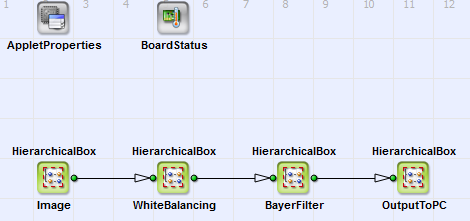Default Platform: mE5-MA-VCL

Short Description

Bayer De-Mosaicing Algorithm According to Laroche

According to Laroche et al. [Lar94] the red, green and blue pixel values from a Bayer-Pattern [Bay76] can be extrapolated using the following algorithm:

##### Interpolation Step 1

In a first step all green pixel values (luminance values) are extrapolated with horizontal and vertical chrominance gradients ( and ). The gradients are defined using a kernel:

Equation 8.

is the chrominance value (red or blue) on the pixel where the green value has to be interpolated. Green is then on the red and blue pixel positions:

Equation 9.

##### Interpolation Step 2

From the interpolated luminance the missing red and blue pixel values can be calculated [Lar94]. Blue on a red pixel or red on a blue pixel:

Equation 10.

blue on a green pixel in a red line or red on a green pixel in a blue line:

Equation 11.

and blue on a green pixel in a blue line and red on a green pixel in a red line:

Equation 12.

Here is the color value red or blue, that has to be determined. The red, green and blue values on the red, green and blue pixels are the ones from the Bayer-Pattern input image.

##### VisualApplets-Design

The complete algorithm (\examples\Processing\Color\Bayer\Laroche_original_maVCL.va) is implemented in a VisualApplets design for a microEnable5 frame grabber (marathon, Lightbride or ironman). You can see its basic design structure in Figure 241, 'Basic design structure'. The content of the HierarchicalBoxes Image and OutputToPC is equivalent to the basic acquisition designs, see e.g. 'Basic Acquisition Examples for Camera Link Cameras for marathon, LightBridge and ironman Frame Grabbers'. In the HierarchicalBox WhiteBalancing a white balancing equivalent to the example in 'Bayer 5x5 De-Mosacing with White Balancing' is performed. In Figure 242, 'Interpolation step 1 of the Bayer-demosaicing process' to Figure 244, 'Content of the HierarchicalBox BlueAndRed' the content of box BayerFilter with detailed comments is shown: In Figure 242, 'Interpolation step 1 of the Bayer-demosaicing process' the gradients and are calculated according to Equation 8. The green values on red and blue pixels are determined according to Equation 9.Figure 241. Basic design structure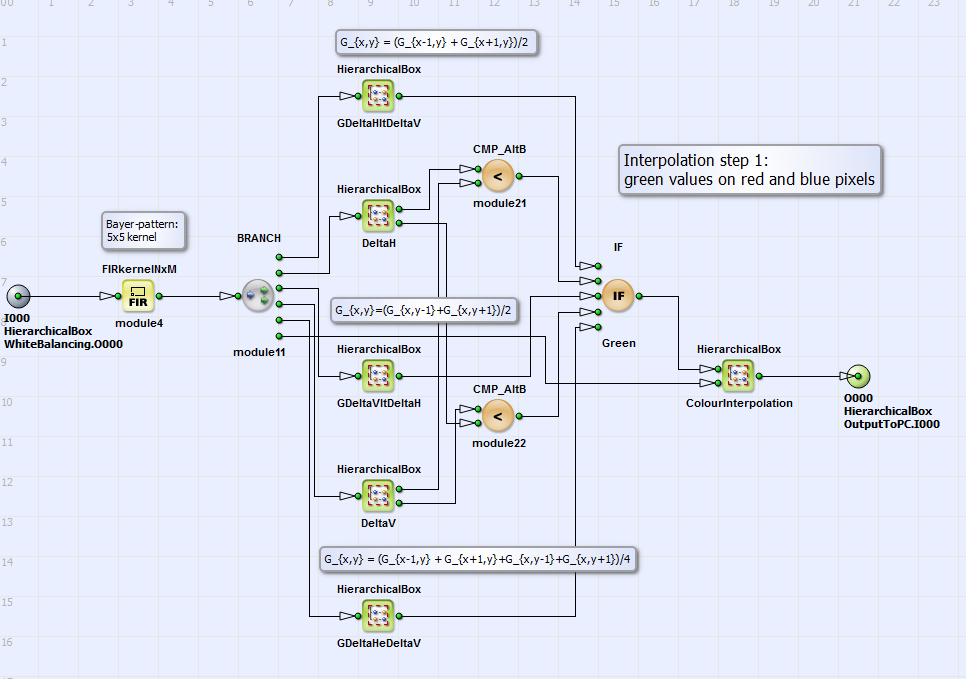Figure 242. Interpolation step 1 of the Bayer-demosaicing process

In Figure 243, 'Content of ColourInterpolation' the content of the HierarchicalBox ColourInterpolation is displayed: In the HierarchicalBox GreenOut the green values of interpolation step 1 are determined as output green values if the current position is a red or blue pixel. Otherwise, if the current position is a green pixel, the output green value is the input green value from the Bayer-pattern. The position determination takes place in WhereAmI RedOrBlue using Modulo-,AND-, NOT and NotEqual-operators. In the HierarchicalBox BlueAndRed all red and blue values are interpolated from the input Bayer-pattern (using a kernel) and the interpolated green values according to Equation 9. In Figure 244, 'Content of the HierarchicalBox BlueAndRed' you can see the content of the hierarchical box BlueAndRed with the interpolation step 2. The synchronization is performed not for a kernel but for a sum of kernel values to save resources on the FPGA. Finally (see Figure 243, 'Content of ColourInterpolation') with the IF-operators RedOut and BlueOut the calculated red and blue values are assigned to the corresponding pixel positions, which are determined in the HierarchicalBox WhereAmIColour (analog to WhereAmI RedOrBlue).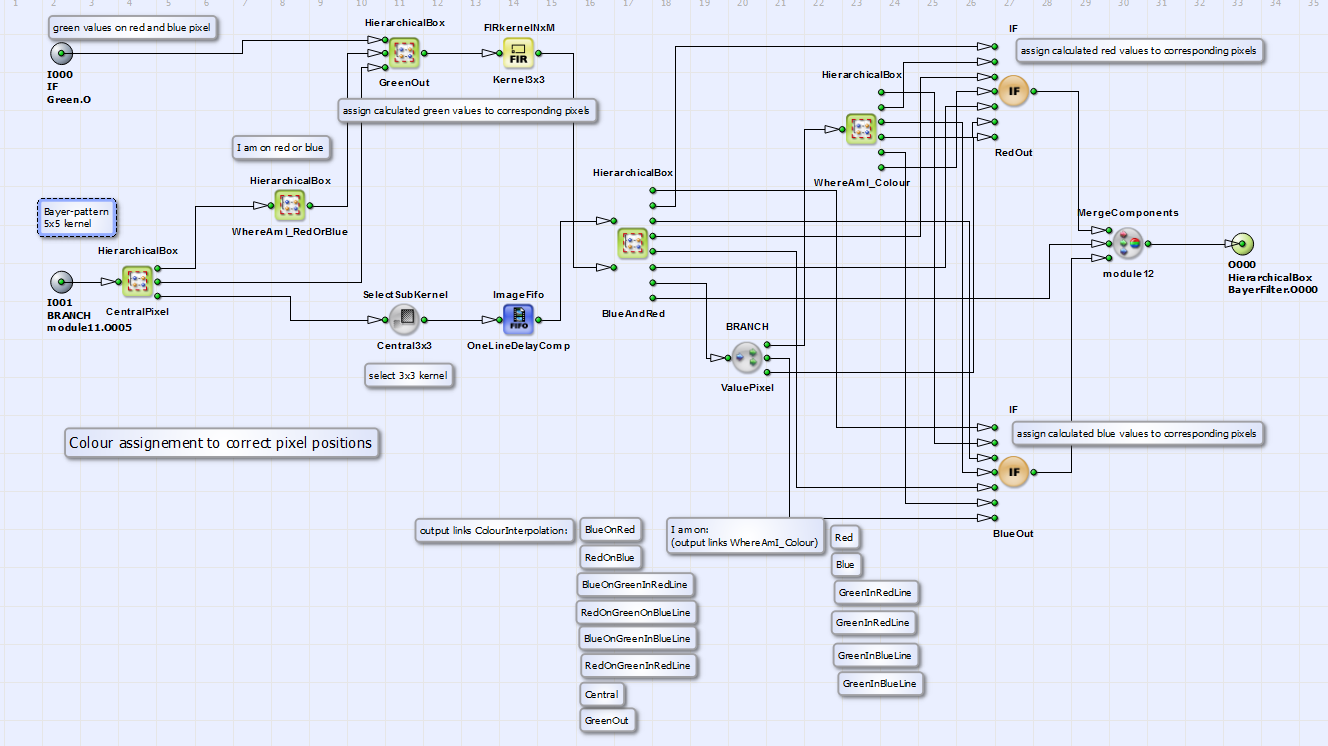Figure 243. Content of ColourInterpolation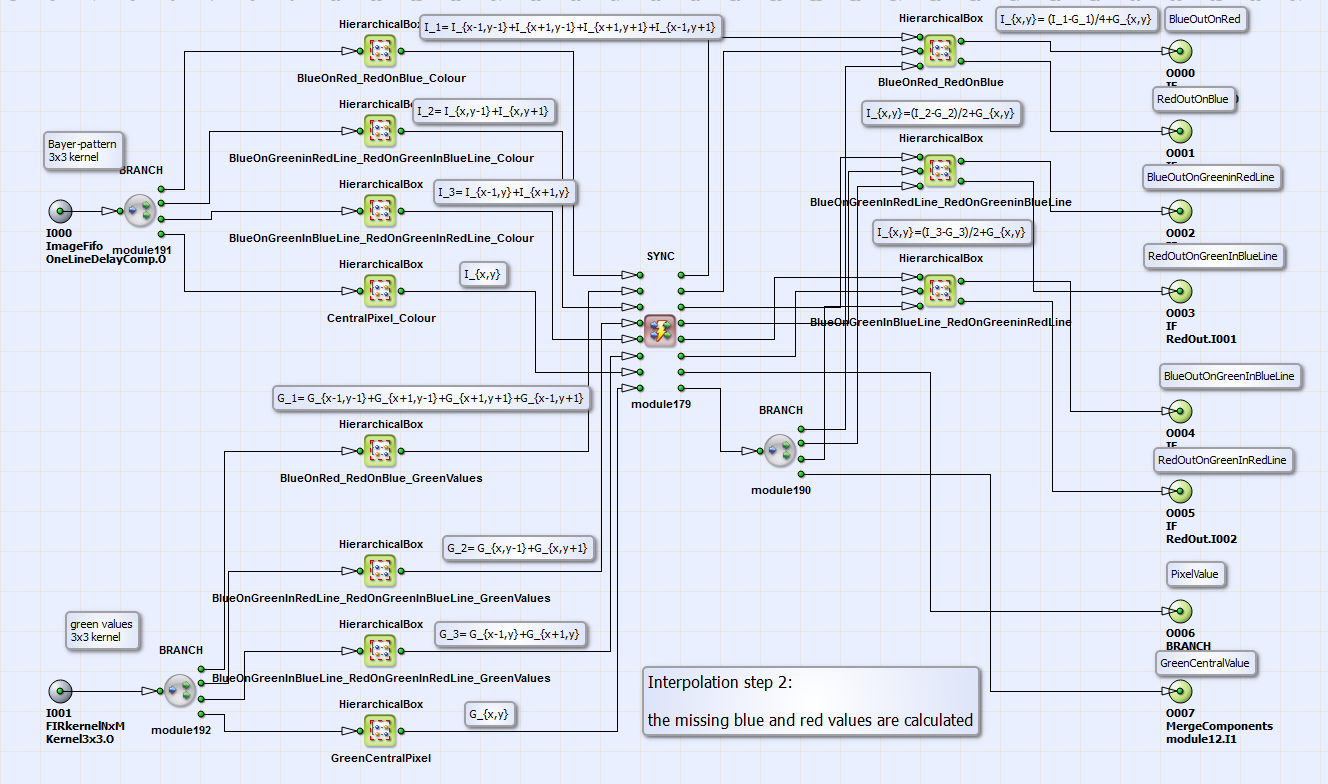Figure 244. Content of the HierarchicalBox BlueAndRed

##### Result of the Bayer-Demosaicing Process

In Figure 246, 'Image demosaiced with the algorithm of Laroche et al. [Lar94]' you can see the result of the Bayer-demosaicing process with the implemented algorithm in comparison to the original artificial color image (Figure 245, 'Original color image') and an image demosaiced with an bilinear interpolation algorithm, using a VA-standard operator Bayer5x5 (Figure 247, 'Image demosaiced with an bilinear algorithm').Figure 245. Original color image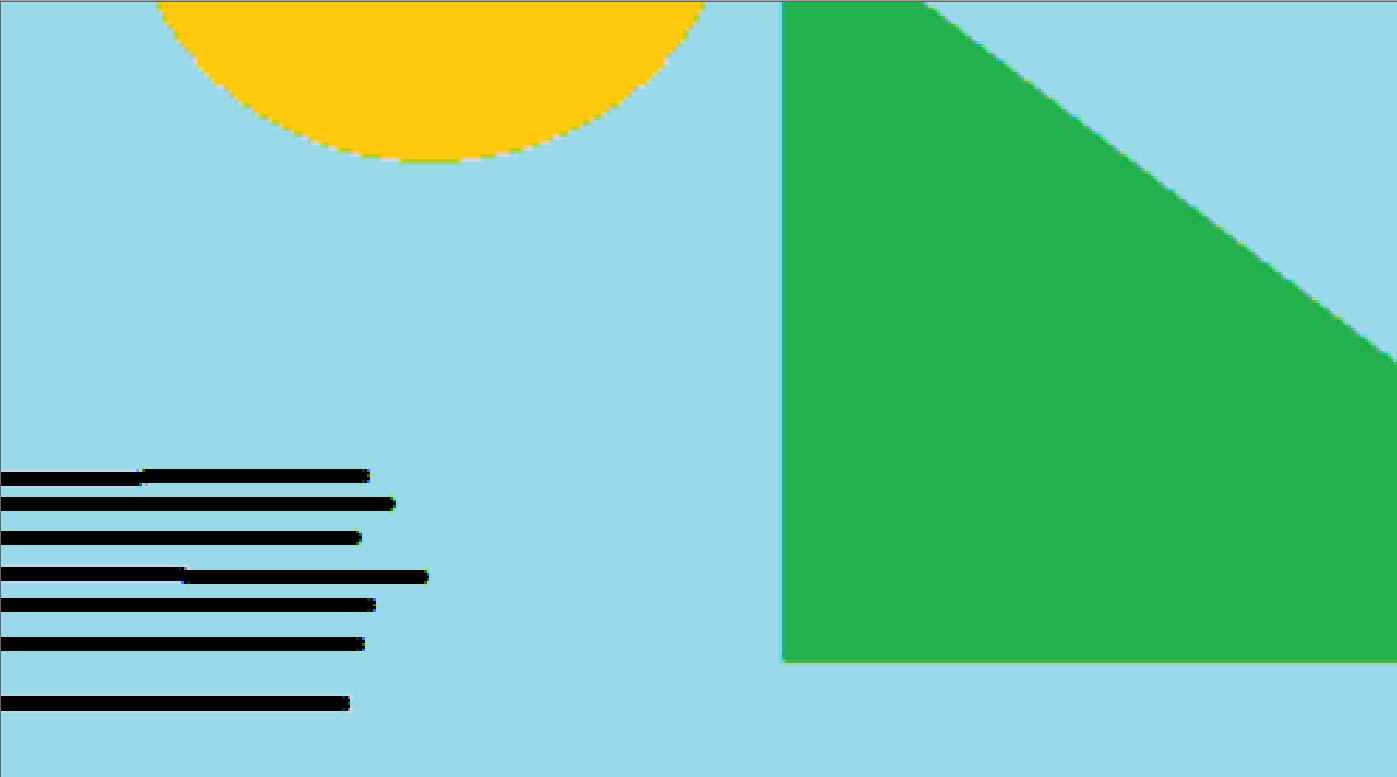Figure 246. Image demosaiced with the algorithm of Laroche et al. [Lar94]

As a result you can see a reduced zipper effect and reduced false color artefacts in comparison to the interpolation method.